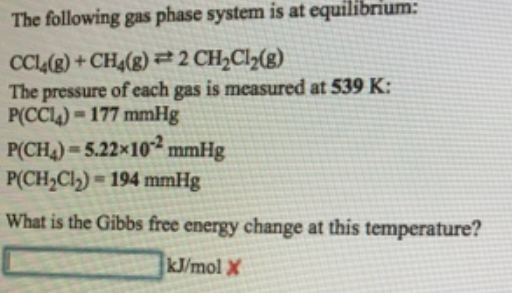# Problem: The following gas phase system is at equilibrium:CCl4(g) + CH4(g)⇌ 2 CH2Cl2(g)The pressure of each gas is measured at 539 K:P(CCl4) = 177 mmHgP(CH4) = 5.22 x 10-2 mmHgP(CH2Cl2) = 194 mmHgWhat is the Gibbs free energy change at this temperature?

###### FREE Expert Solution
91% (289 ratings)###### Problem Details

The following gas phase system is at equilibrium:

CCl4(g) + CH4(g)⇌ 2 CH2Cl2(g)

The pressure of each gas is measured at 539 K:

P(CCl4) = 177 mmHg
P(CH4) = 5.22 x 10-2 mmHg
P(CH2Cl2) = 194 mmHg

What is the Gibbs free energy change at this temperature?